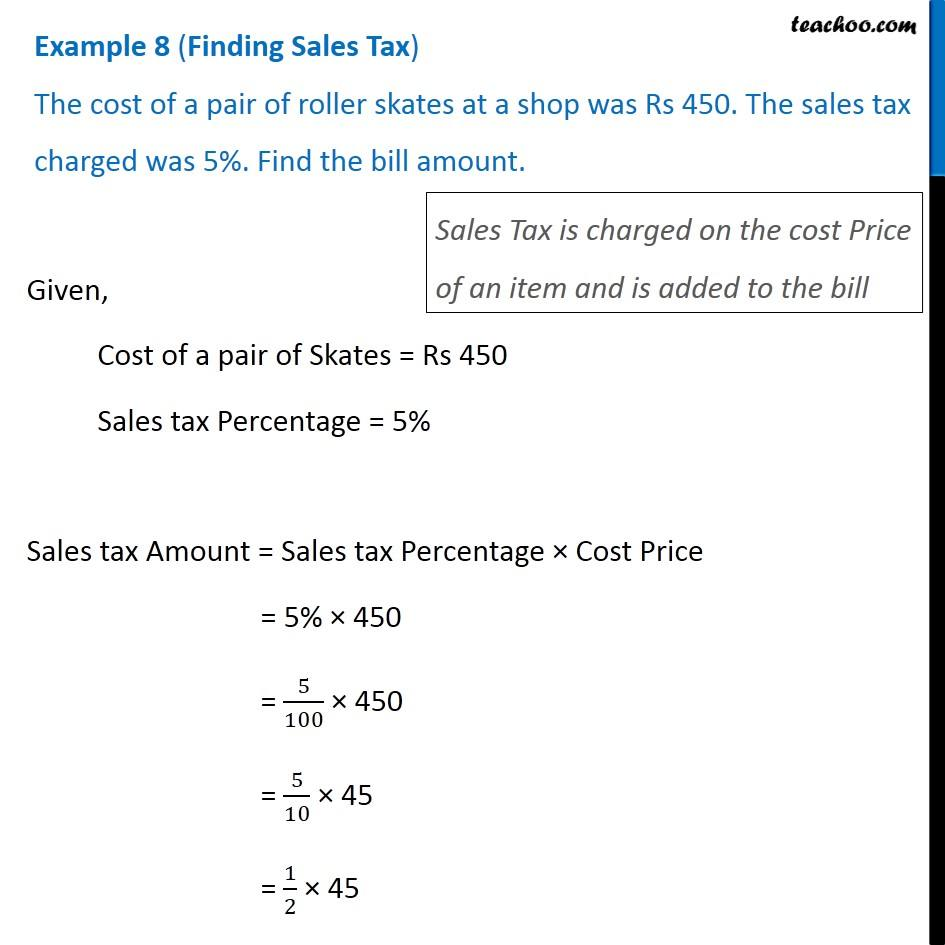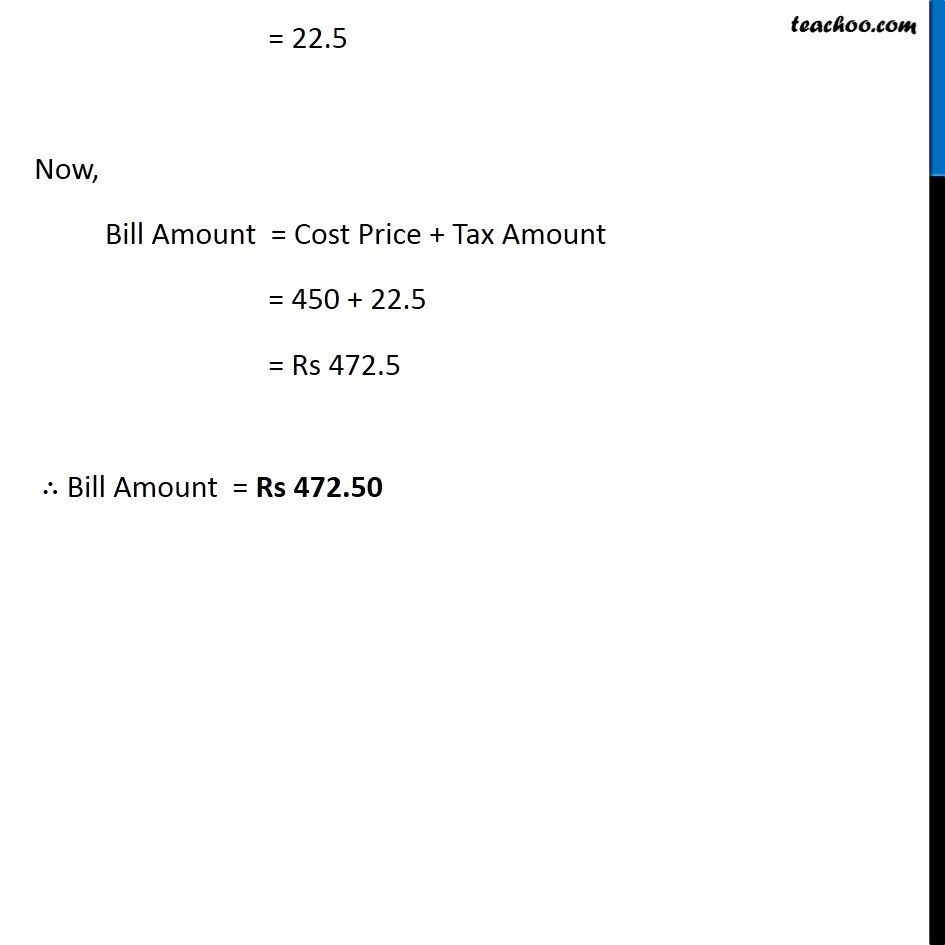Examples

Chapter 8 Class 8 Comparing Quantities
Serial order wiseGet live Maths 1-on-1 Classs - Class 6 to 12

### Transcript

Example 8 (Finding Sales Tax) The cost of a pair of roller skates at a shop was Rs 450. The sales tax charged was 5%. Find the bill amount. Sales Tax is charged on the cost Price of an item and is added to the bill Given, Cost of a pair of Skates = Rs 450 Sales tax Percentage = 5% Sales tax Amount = Sales tax Percentage × Cost Price = 5% × 450 = 5/100 × 450 = 5/10 × 45 = 1/2 × 45 = 22.5 Now, Bill Amount = Cost Price + Tax Amount = 450 + 22.5 = Rs 472.5 ∴ Bill Amount = Rs 472.50